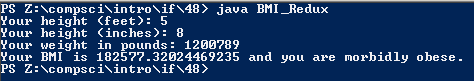# Assignment 48; BMI_Redux

## Code

```    // Donovan Rich
// 6
// BMICalculator
// BMICalculator.java
// 11/4/2015

import java.util.Scanner;

public class BMI_Redux {

public static void main( String[] args ) {

Scanner keyboard = new Scanner(System.in);
double m, kg, lbs, in, ft, bmi;
String category="N/A";

System.out.print( "Your height (feet): " );
ft = keyboard.nextDouble();

System.out.print( "Your height (inches): " );
in = keyboard.nextDouble();

System.out.print( "Your weight in pounds: " );
lbs = keyboard.nextDouble();

kg = (lbs * 0.453592);
m = (in * 0.0254) + (ft * 0.3048);

bmi = kg / (m*m);

if ( bmi < 15.0 )
{
category = "very severely underweight";
}
if ( bmi >= 15.0 && bmi <= 16.0 )
{
category = "severely underweight";
}
if ( bmi > 16.0 && bmi <= 18.4 )
{
category = "underweight";
}
if ( bmi > 18.4 && bmi <= 24.9 )
{
category = "of a normal weight";
}
if ( bmi > 24.9 && bmi <= 29.9 )
{
category = "overweight";
}
if ( bmi > 29.9 && bmi <= 34.9 )
{
category = "moderately obese";
}
if ( bmi > 34.9 && bmi <= 39.9 )
{
category = "severely obese";
}
if ( bmi > 39.9 )
{
category = "morbidly obese";
}

System.out.println( "Your BMI is " + bmi + " and you are " + category + "." );
}
}

```

### Picture of the output